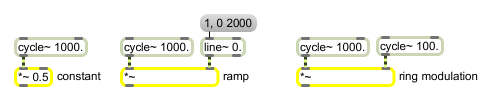# *~

Multiply two signals

## Description

*~ is a signal multiplier-operator that outputs a signal which is the multiplication between two signals.

## Arguments

Name Type Opt Description
initial-multiplier float or int opt Sets an initial value by which to multiply the signal coming into the left inlet. If a signal is connected to the right inlet, the argument is ignored. If no argument is present, and no signal is connected to the right inlet, the initial value is 0 by default.

## Messages

 int input [int] In left inlet: A factor by which to multiply the signal coming into the right inlet. If a signal is also connected to the left inlet, a float or int is ignored. In right inlet: A factor by which to multiply the signal coming into the left inlet. If a signal is also connected to the right inlet, a float or int is ignored. float input [float] In left inlet: A factor by which to multiply the signal coming into the right inlet. If a signal is also connected to the left inlet, a float or int is ignored. In right inlet: A factor by which to multiply the signal coming into the left inlet. If a signal is also connected to the right inlet, a float or int is ignored. signal In left inlet: The signal is multiplied by the signal coming into the right inlet, or a constant value received in the right inlet. In right inlet: The signal is multiplied by the signal coming into the left inlet, or a constant value received in the left inlet.

## Output

signal: The product of the two inputs.

## ExamplesScale a signal's amplitude by a constant or changing value or by another audio signal